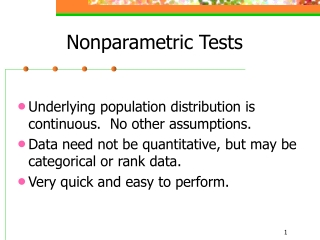DownloadDownload PresentationNonparametric Tests

Nonparametric Tests

Télécharger la présentationNonparametric Tests

- - - - - - - - - - - - - - - - - - - - - - - - - - - E N D - - - - - - - - - - - - - - - - - - - - - - - - - - -
Presentation Transcript

1. Nonparametric Tests • Underlying population distribution is continuous. No other assumptions. • Data need not be quantitative, but may be categorical or rank data. • Very quick and easy to perform.

2. Parametric vs. Nonparametric • When distribution is normal, parametric test is better. The nonparametric test requires larger sample size to achieve same power. • When distribution is not close to normal, nonparametric methods are much better. • Choose the parametric procedure whenever possible.

3. + - + - Sign Test (1) • Tests hypotheses about the median. • Null hypothesis H0: • Find the differences xi – • Test statistic: R+ is number of differences that are positive. • What are the P-values for different tests? • Reject H0 if the P-value is less than a.

4. + - + - Sign Test (2) • When n is large (at least 10), and p=0.5, the binomial is approximately normal. • R+ has a normal distribution with mean 0.5n and variance 0.25n. • The test statistic is:

5. + - + - Sign Test for Paired Samples • Dj = X1j – X2j • The test statistic is:

6. + - + - Type II Error • In determining b, we need: • The alternative median • AND the form of the underlying distribution

7. + - + - Comparison to the t-Test • If underlying population is normal • Either test can be used • T-test has smallest b for all one-sided tests • T-test has smallest b for all two-sided tests with symmetric critical regions • If underlying population is nonnormal but symmetric • T-test will have smaller b unless tails are heavy • Sign test is considered test for median, rather than serious competitor for the t-test.